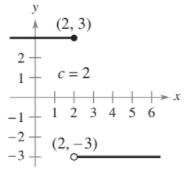×
Get Full Access to Calculus: Early Transcendental Functions - 6 Edition - Chapter 2.4 - Problem 5
Get Full Access to Calculus: Early Transcendental Functions - 6 Edition - Chapter 2.4 - Problem 5

×

# ?Limits and Continuity In Exercises 1-6, use the graph to determine the limit, and discuss the continuity of the function. (a) $$\lim _{x \rigISBN: 9781285774770 141 ## Solution for problem 5 Chapter 2.4 Calculus: Early Transcendental Functions | 6th Edition • Textbook Solutions • 2901 Step-by-step solutions solved by professors and subject experts • Get 24/7 help from StudySoup virtual teaching assistantsCalculus: Early Transcendental Functions | 6th Edition 4 5 1 265 Reviews 19 1 Problem 5 Limits and Continuity In Exercises 1-6, use the graph to determine the limit, and discuss the continuity of the function. (a) \(\lim _{x \rightarrow c^{+}} f(x)$$

(b) $$\lim _{x \rightarrow c^{-}} f(x)$$

(c) $$\lim _{x \rightarrow c} f(x)$$ lim_x rightarrow c f(x)Text Transcription:

lim_x rightarrow c^+ f(x)

lim_x rightarrow c^- f(x)

lim_x rightarrow c f(x)

Step-by-Step Solution:

Step 1 of 5) Figure 8.4 shows the region whose area is represented by this integral.

Step 2 of 2

## Discover and learn what students are asking

Calculus: Early Transcendental Functions : Change of Variables: Polar Coordinates
?Describing a Region In Exercises 5-8, use polar coordinates to describe the region shown.

Statistics: Informed Decisions Using Data : Tests for Independence and the Homogeneity of Proportions
?Dropping a Course A survey was conducted at a community college of 50 randomly selected students who dropped a course in the current semester to learn

Unlock Textbook Solution